Stress Distribution in the Soil

# Stress Distribution in the Soil - Notes | Study Civil Engineering SSC JE (Technical) - Civil Engineering (CE)

 1 Crore+ students have signed up on EduRev. Have you?

Chapter 8
Stress Distribution in the Soil

VERTICAL STRESS:
Stress is induced in a soil mass due to weight  of overlying soil and due to applied load. This is called vertical stress. Stress in the soil may be caused by:
1. Self weight of soil

BOUSSINESQ’S THEORY
Assumption:
1. Soil mass is homogeneous, elastic and isotropic
2. Soil mass is semi-infinite (Surface in infinite but depth to finite)
3. Soil is weight less unstressed before application of load. (Self weight of soil is ignored)
4. No change in volume of soil takes place due to applical of the loads.
Boussinesq (1985) evolved equations that can be used to determine stresses at any point P at a depth z as a result of a surface point load.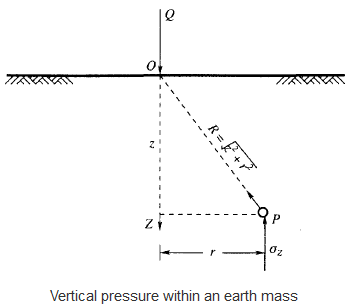At point P of above figure due to a point load Q,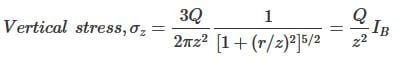where,
r = the horizontal distance between an arbitrary point P below the surface and the vertical axis through the point load Q.
z = the vertical depth of the point P from the surface.
IB=Boussinesq stress coefficient =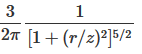This intensity of vertical stress directly below the point load, on its axis of loading (r=0) is given by: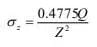Boussinesq's Result: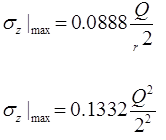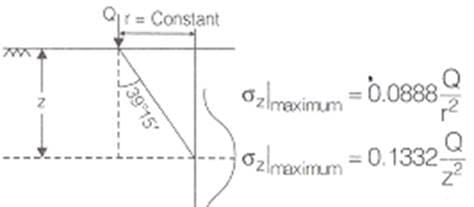WESTERGAARD’S THEORY:

Assumptions:
(1) The soil is elastic and semi-infinite.
(2) Soil is composed of numerous closely spaced horizontal layers of negligible thickness of an infinite rigid material.
(3) The rigid material permits only the downward deformation of mass in which horizontal deformation is zero.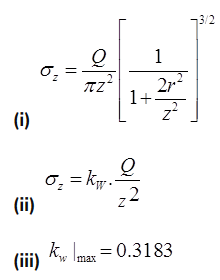NOTE: Boussinesq’s theory is applicable for Isotropic soil where as westergaards theory is applicable for non- Isotropic soil.

WESTERGAARDS RESULTS
(i) Vertical Stress due to Live Loads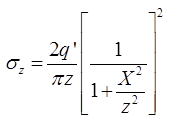where, σz = Vertical stress of any point having coordinate (x, z)
at X = 0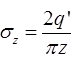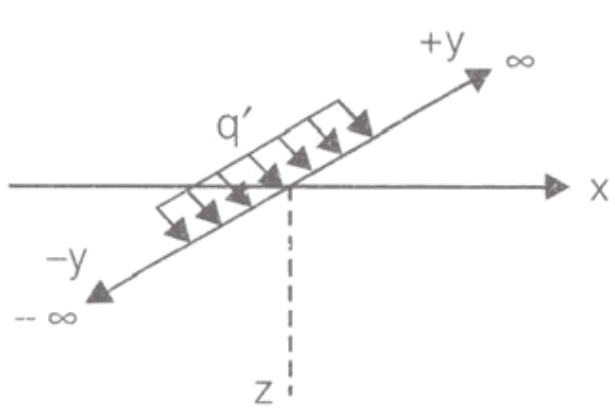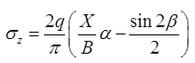where,  σz = Vertical stress at point ‘p’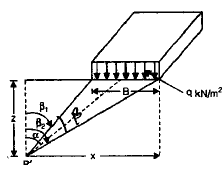(iii)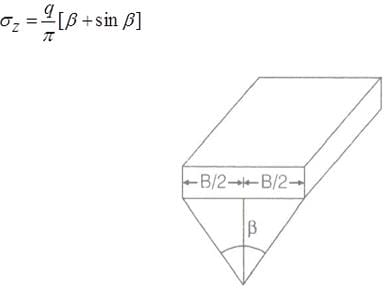(iv) Vertical stress below uniform load acting on a circular area.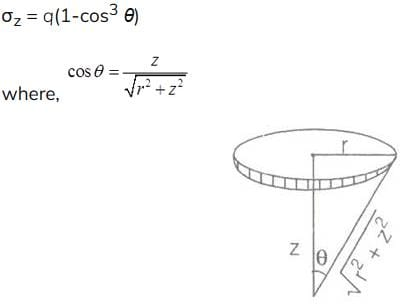NEWMARKS’S CHART METHOD:
This method is applicable to semi-infinite, homogeneous, isotropic and elastic soil mass. It is not applicable for layered structure. The greatest advantage of this method is that it can be applied for uniformly distributed area of irregular shape. Newmark’s chart is made of concentric circles and radial lines. Normally there are 10 concentric circles and 20 radial lines.
No.of concentric circle =10
Influence of area (1)=influence of area(2)=Influence of area(3)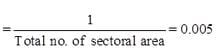σz = 0.005qNa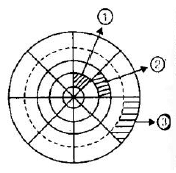where, NA = Total number of sectorial area of Newmark’s chart.

APPROXIMATE METHOD: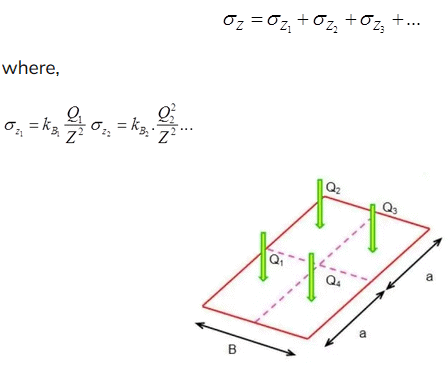(ii) Trapezoidal Method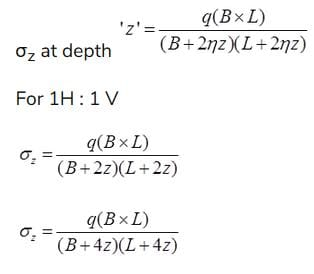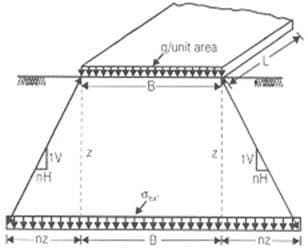(iii) Stress Isobar method : Area Bounded by 0.2q stress isobar is considered to be stressed by vertical stress on loading.

0.2q =20%Stress Isobar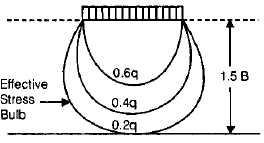The document Stress Distribution in the Soil - Notes | Study Civil Engineering SSC JE (Technical) - Civil Engineering (CE) is a part of the Civil Engineering (CE) Course Civil Engineering SSC JE (Technical).
All you need of Civil Engineering (CE) at this link: Civil Engineering (CE)

## Civil Engineering SSC JE (Technical)

2 videos|122 docs|50 tests
 Use Code STAYHOME200 and get INR 200 additional OFF

## Civil Engineering SSC JE (Technical)

2 videos|122 docs|50 tests

Track your progress, build streaks, highlight & save important lessons and more!

,

,

,

,

,

,

,

,

,

,

,

,

,

,

,

,

,

,

,

,

,

;